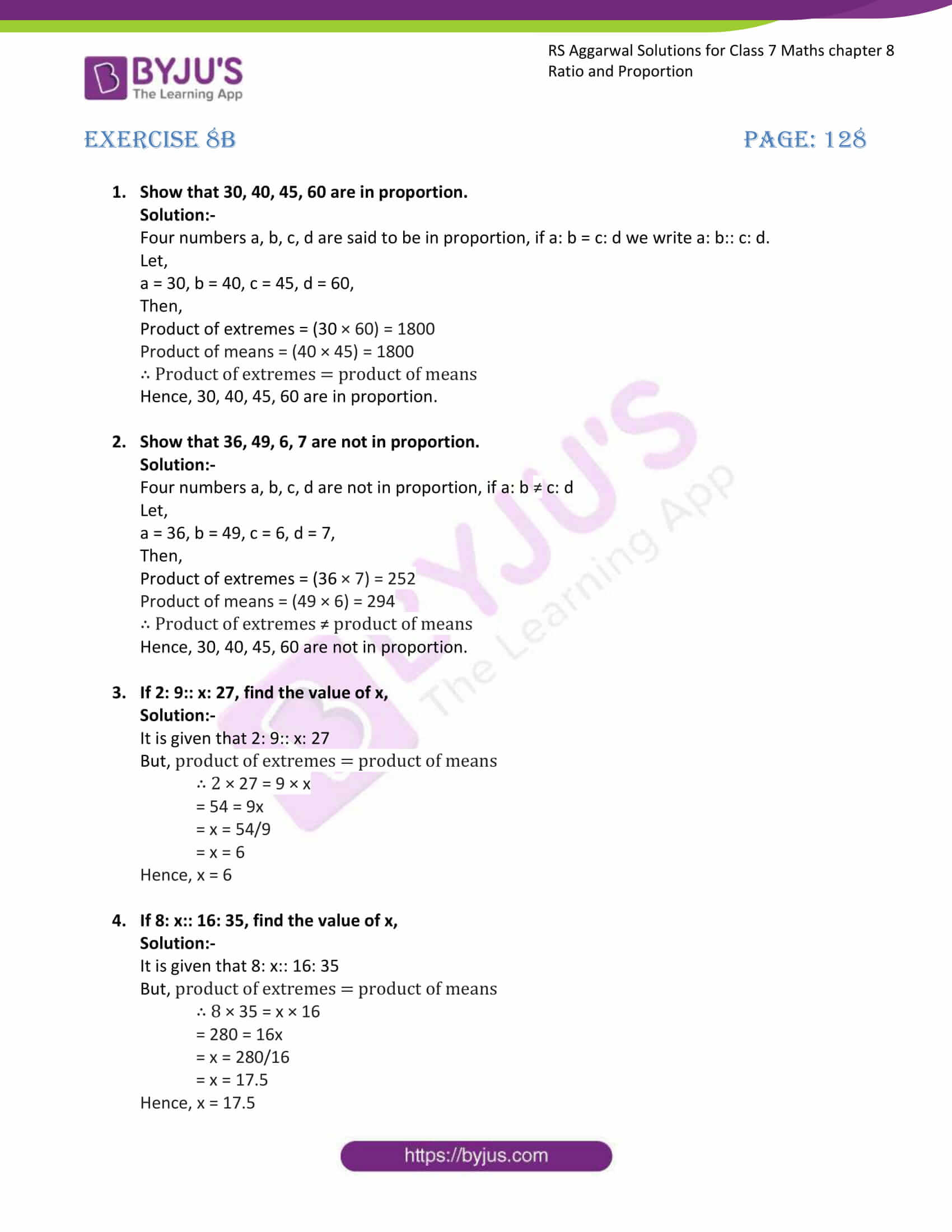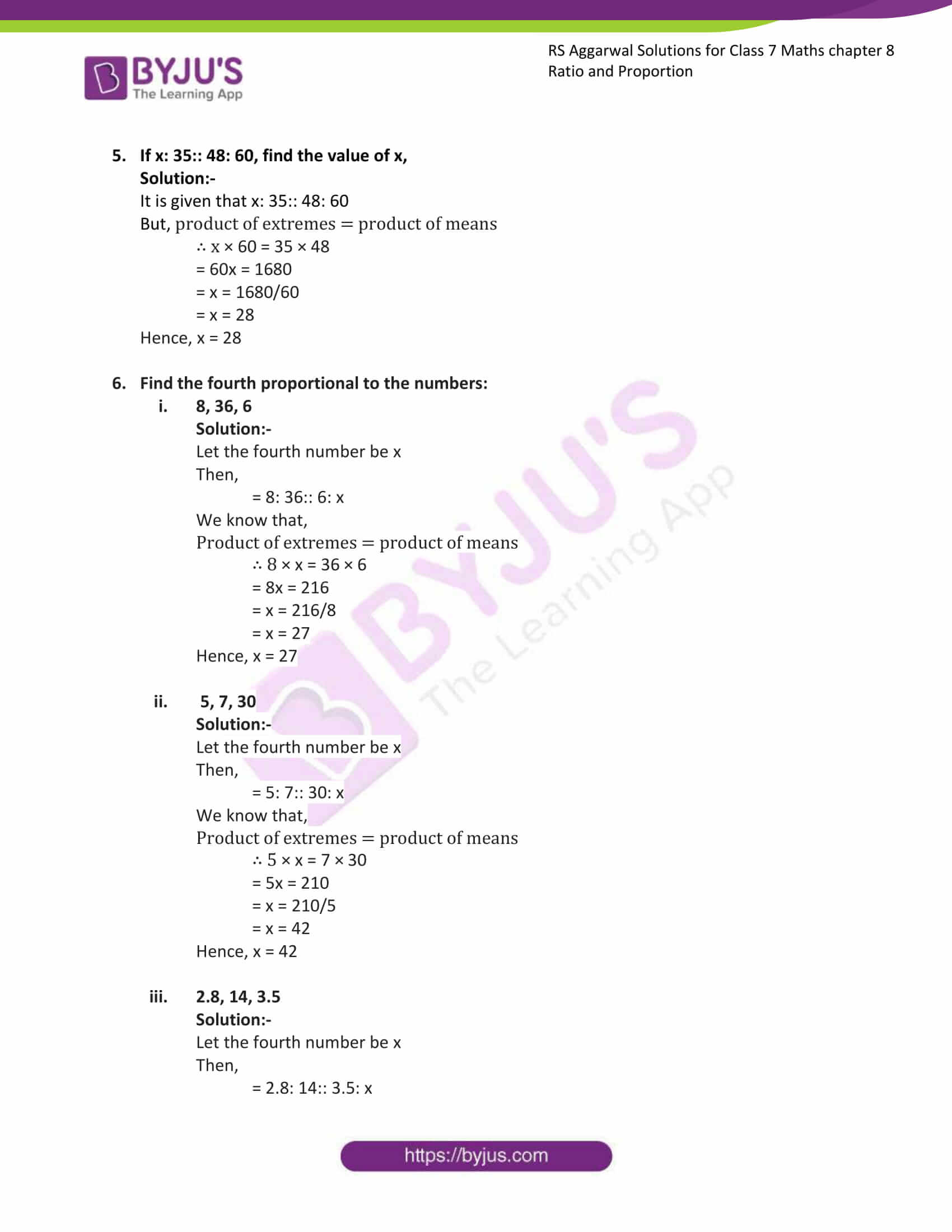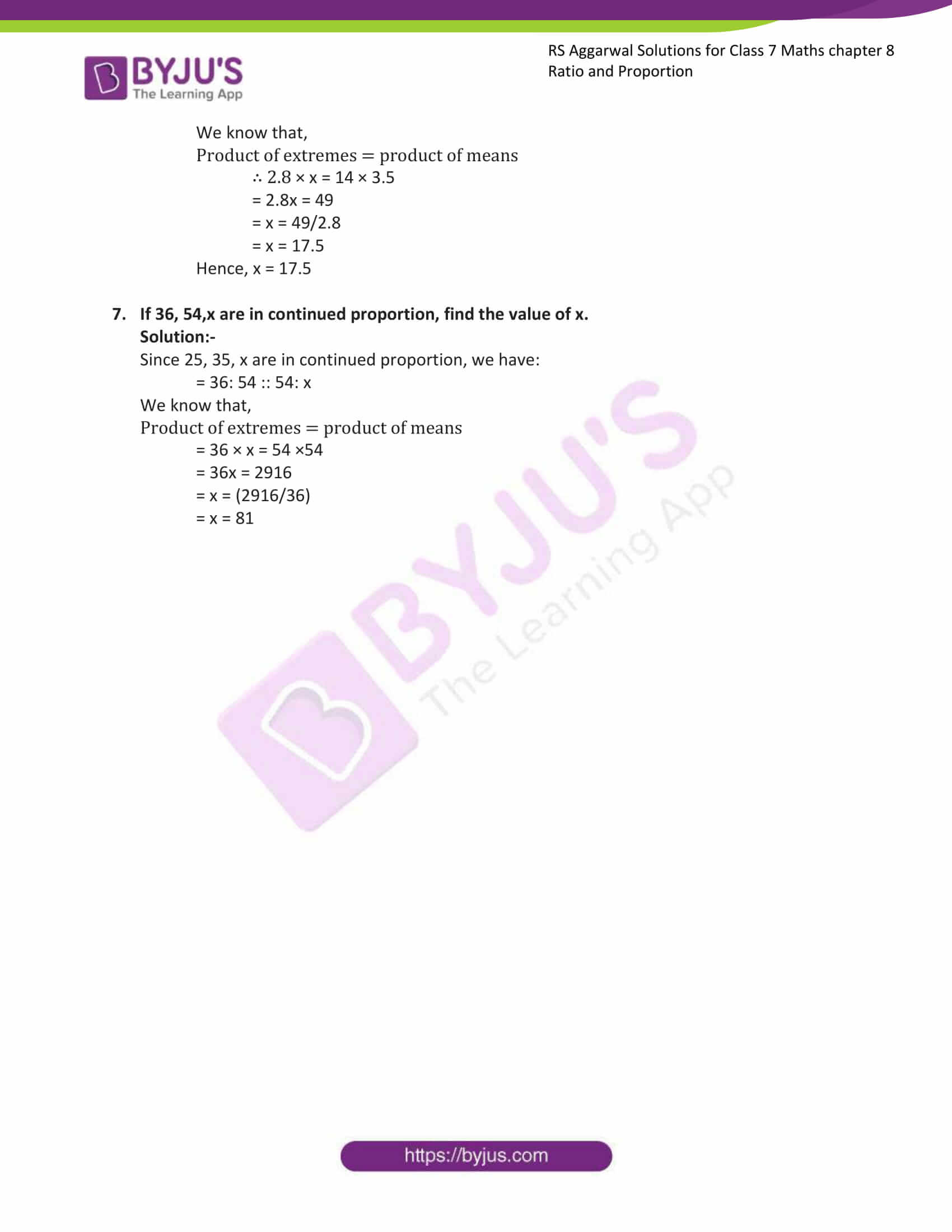# RS Aggarwal Solutions for Class 7 Maths Exercise 8B Chapter 8 Ratio and Proportion

RS Aggarwal Solutions for Class 7 Maths Exercise 8B Chapter 8 Ratio and Proportion in simple PDF are provided here. This exercise of RS Aggarwal Solutions for Class 7 Chapter 8 contains questions related to the topic Proportion. Four numbers a, b, c, d are said to be in proportion if a : b is equal to c : d. Learn further about these topics by solving the questions of RS Aggarwal Solutions for Class 7 Maths Chapter 8 Ratio and Proportion with the help of solutions given here.

## Download the PDF of RS Aggarwal Solutions For Class 7 Maths Chapter 8 Ratio and Proportion – Exercise 8B### Access answers to Maths RS Aggarwal Solutions for Class 7 Chapter 8 – Ratio and Proportion Exercise 8B

1. Show that 30, 40, 45, 60 are in proportion.

Solution:-

Four numbers a, b, c, d are said to be in proportion, if a: b = c: d we write a: b:: c: d.

Let,

a = 30, b = 40, c = 45, d = 60,

Then,

Product of extremes = (30 × 60) = 1800

Product of means = (40 × 45) = 1800

∴ Product of extremes = product of means

Hence, 30, 40, 45, 60 are in proportion.

2. Show that 36, 49, 6, 7 are not in proportion.

Solution:-

Four numbers a, b, c, d are not in proportion, if a: b ≠ c: d

Let,

a = 36, b = 49, c = 6, d = 7,

Then,

Product of extremes = (36 × 7) = 252

Product of means = (49 × 6) = 294

∴ Product of extremes ≠ product of means

Hence, 30, 40, 45, 60 are not in proportion.

3. If 2: 9:: x: 27, find the value of x,

Solution:-

It is given that 2: 9:: x: 27

But, product of extremes = product of means

∴ 2 × 27 = 9 × x

= 54 = 9x

= x = 54/9

= x = 6

Hence, x = 6

4. If 8: x:: 16: 35, find the value of x,

Solution:-

It is given that 8: x:: 16: 35

But, product of extremes = product of means

∴ 8 × 35 = x × 16

= 280 = 16x

= x = 280/16

= x = 17.5

Hence, x = 17.5

5. If x: 35:: 48: 60, find the value of x,

Solution:-

It is given that x: 35:: 48: 60

But, product of extremes = product of means

∴ x × 60 = 35 × 48

= 60x = 1680

= x = 1680/60

= x = 28

Hence, x = 28

6. Find the fourth proportional to the numbers:

1. 8, 36, 6

Solution:-

Let the fourth number be x

Then,

= 8: 36:: 6: x

We know that,

Product of extremes = product of means

∴ 8 × x = 36 × 6

= 8x = 216

= x = 216/8

= x = 27

Hence, x = 27

2. 5, 7, 30

Solution:-

Let the fourth number be x

Then,

= 5: 7:: 30: x

We know that,

Product of extremes = product of means

∴ 5 × x = 7 × 30

= 5x = 210

= x = 210/5

= x = 42

Hence, x = 42

3. 2.8, 14, 3.5

Solution:-

Let the fourth number be x

Then,

= 2.8: 14:: 3.5: x

We know that,

Product of extremes = product of means

∴ 2.8 × x = 14 × 3.5

= 2.8x = 49

= x = 49/2.8

= x = 17.5

Hence, x = 17.5

4. If 36, 54,x are in continued proportion, find the value of x.

Solution:-

Since 25, 35, x are in continued proportion, we have:

= 36: 54 :: 54: x

We know that,

Product of extremes = product of means

= 36 × x = 54 ×54

= 36x = 2916

= x = (2916/36)

= x = 81

### Access other exercises of RS Aggarwal Solutions For Class 7 Chapter 8 – Ratio and Proportion

Exercise 8C Solutions